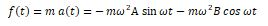The International Information Center for Structural Engineers

Tuesday, 12 June 2018 11:45cat

## Calculation Example – Simple harmonic vibration part 2 Featured

Written by  TheStructuralEngineer.info

A single point with mass m is vibrating harmonically. The displacement x from point of balance is governed by the differential equationwhere ω is a positive constant.

b) What is the speed, the acceleration, and the force at the mass as a function of time t and constants A, B.

Solution

The derivative of x(t)=A sin⁡ωt+B cos⁡ ωt

is the speed       u(t)=x'(t)=Aω cos⁡ωt-B ωsin⁡ωt

And the derivative of speed is the acceleration

a(t)=u'(t)=x''(t)=-〖ω^2 Α sin〗⁡ωt-ω^2 B cos⁡ωt

The force at the mass isvideo

• Default
• Title
• Date
• Random
• ## Keller lays foundation for Aston Martin tower

Keller business units HJ Foundation and Hayward Baker worked on the foundation system of one of Miami's most prestigious, eye-catching